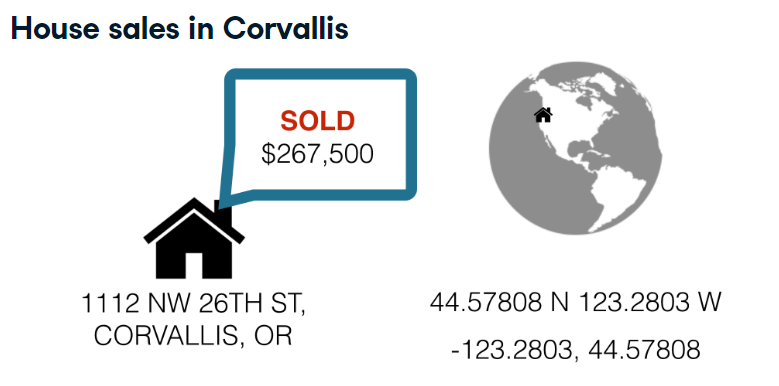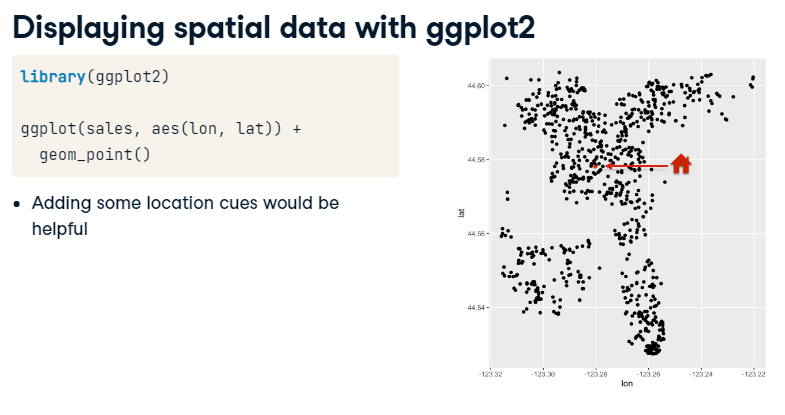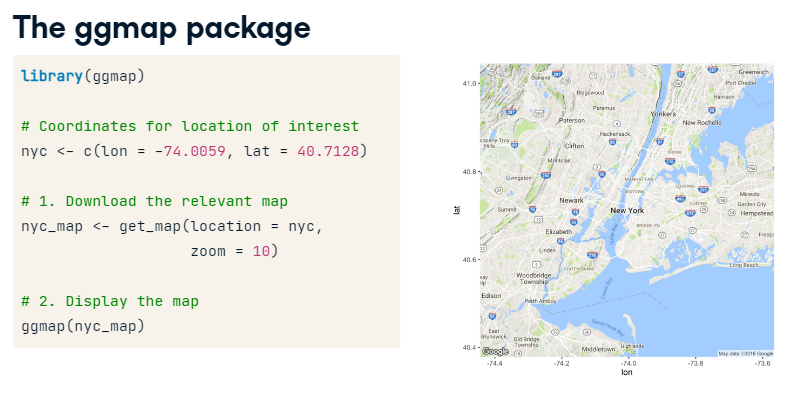What is spatial data?

• data associated with locations
• location described by coordinates + a coordinate reference system (CRS)
• common CRS: longitude, latitude describes locations on the surface of the EarthPoint data: locations are points, described by a single pair of coordinatesGrabbing a background map

There are two steps to adding a map to a `ggplot2` plot with `ggmap`:

1. Download a map using `get_map()`
2. Display the map using `ggmap()`

As an example, let’s grab a map for New York City:

``````library(ggmap)

nyc <- c(lon = -74.0059, lat = 40.7128)
nyc_map <- get_map(location = nyc, zoom = 10)``````

`get_map()` has a number of arguments that control what kind of map to get, but for now you’ll mostly stick with the defaults. The most important argument is the first, `location`, where you can provide a longitude and latitude pair of coordinates where you want the map centered. (We found these for NYC from a quick google search of “coordinates nyc”.) The next argument, `zoom`, takes an integer between 3 and 21 and controls how far the mapped is zoomed in. In this exercise, you’ll set a third argument, `scale`, equal to 1. This controls the resolution of the downloaded maps and you’ll set it lower (the default is 2) to reduce how long it takes for the downloads.

Displaying the map is then as simple as calling `ggmap()` with your downloaded map as the only argument: `ggmap(nyc_map)`

Your turn! We are going to be looking at house sales in Corvallis, but you probably have no idea where that is! Let’s find out.

``````library(pacman)